# MSBSHSE Solutions For Class 8 Maths Part 1 Chapter 3- Indices and Cube Root

MSBSHSE Solutions For Class 8 Maths Part 1 Chapter 3 Indices and Cube Root is one of the best reference material students can rely on. The MSBSHSE Solution for Class 8 module contains all the important topics that can help students score well in the examinations. Facilitators have provided the solutions accurately, depending upon the students grasping abilities to understand the concepts clearly. Every question is explained stepwise for a better understanding of the students.

The best reference guide for the students is the Maharashtra State Board Class 8 Textbooks Part 1 Maths Solutions. Students can analyse their weaker section and can improve on it by thoroughly going through the solutions, after completing every chapter. The PDF of Maharashtra Board Solutions for Class 8 Maths Chapter 3 Indices and Cube Root is provided here. Students can refer and download from the given links. This chapter is based on indices and cube root. Some of the essential topics of this chapter includes the meaning of numbers with rational indices, Cube and Cube root.

## Download the PDF of Maharashtra Board Solutions For Class 8 Maths Part 1 Chapter 3- Indices and Cube Root.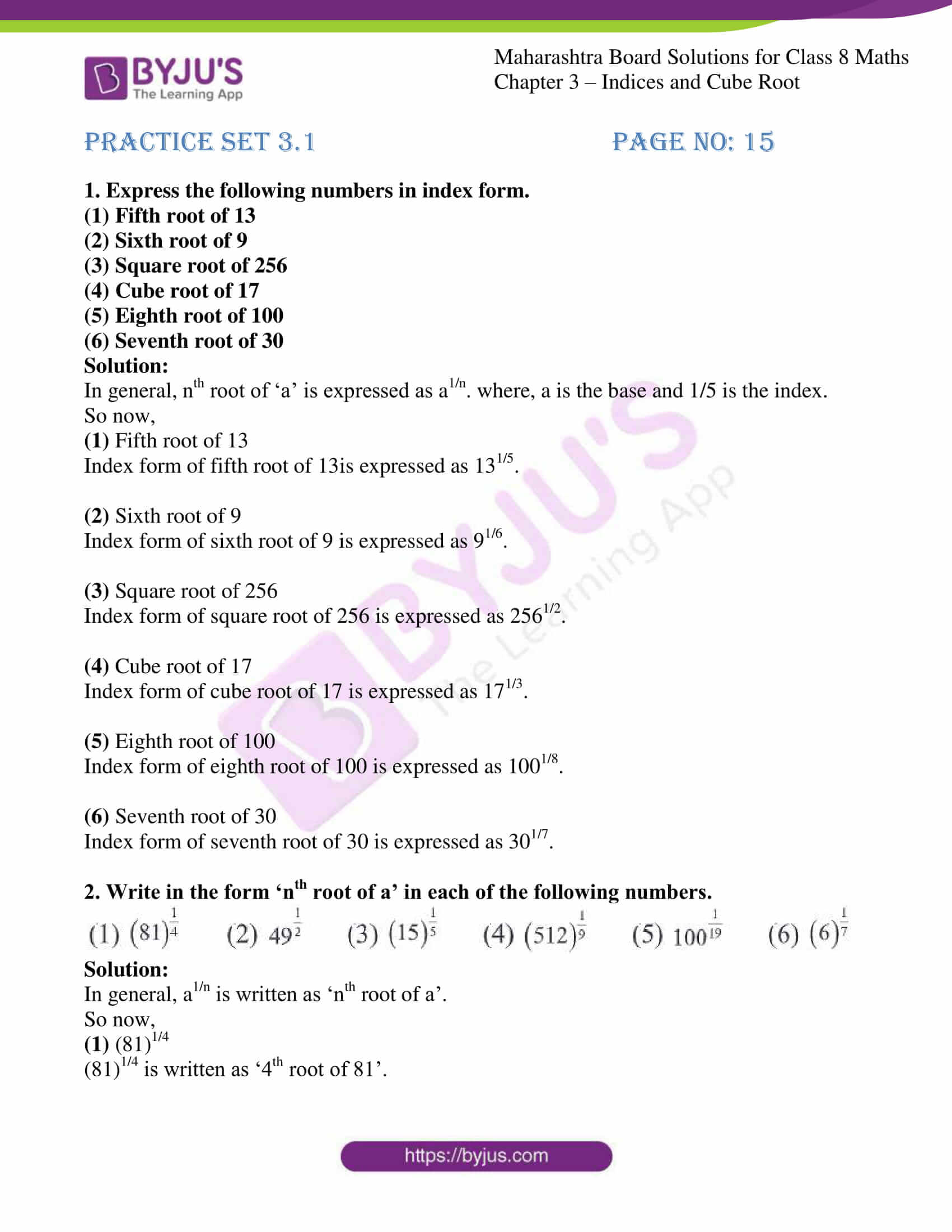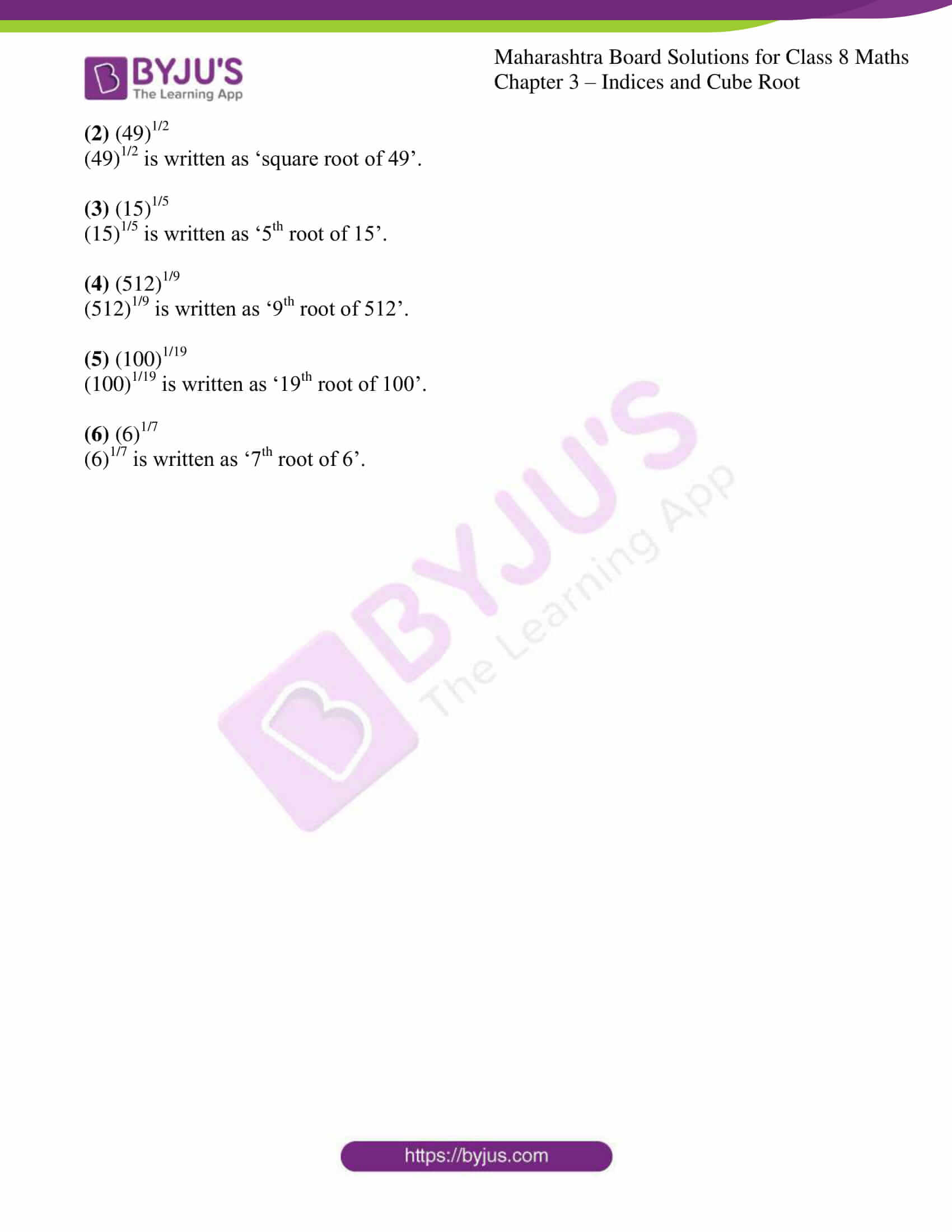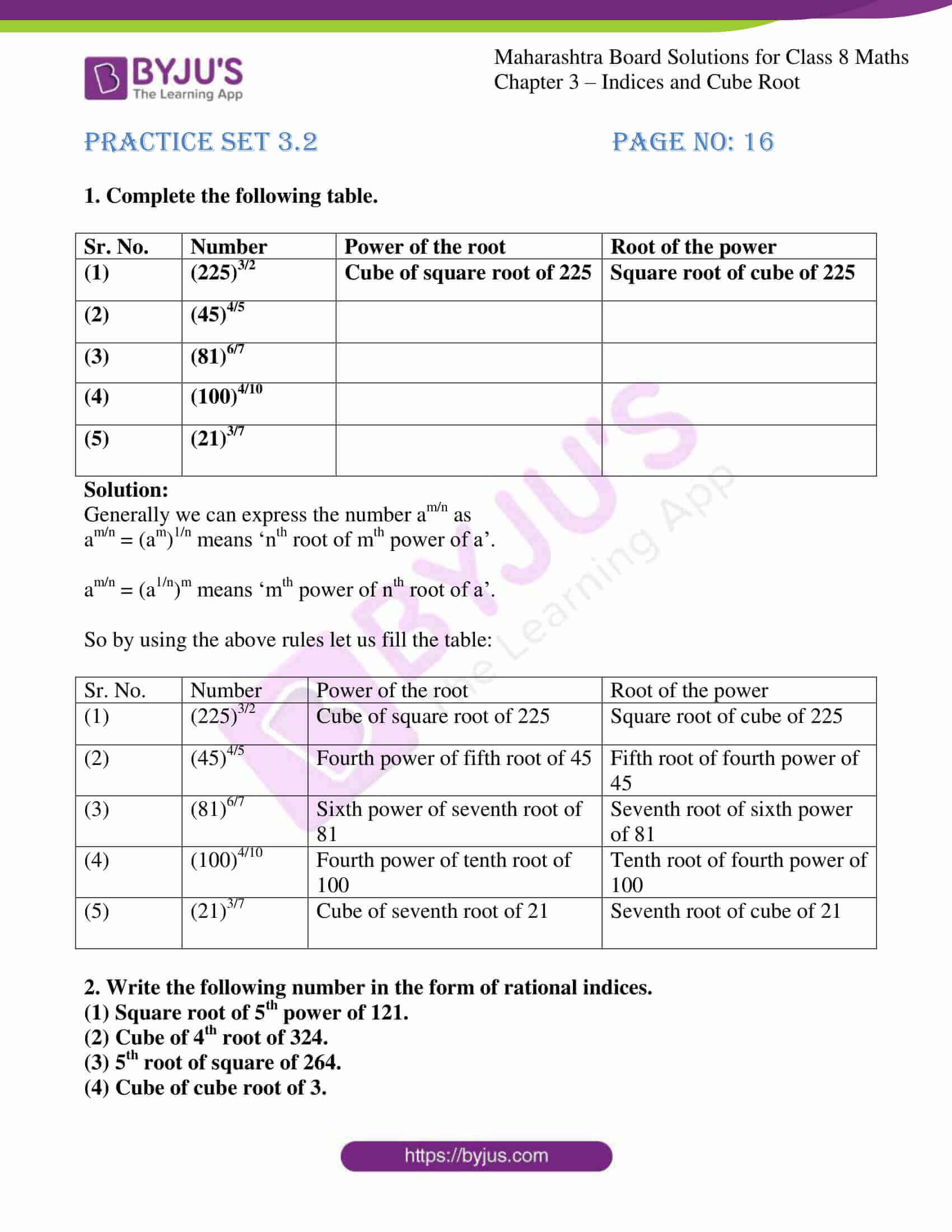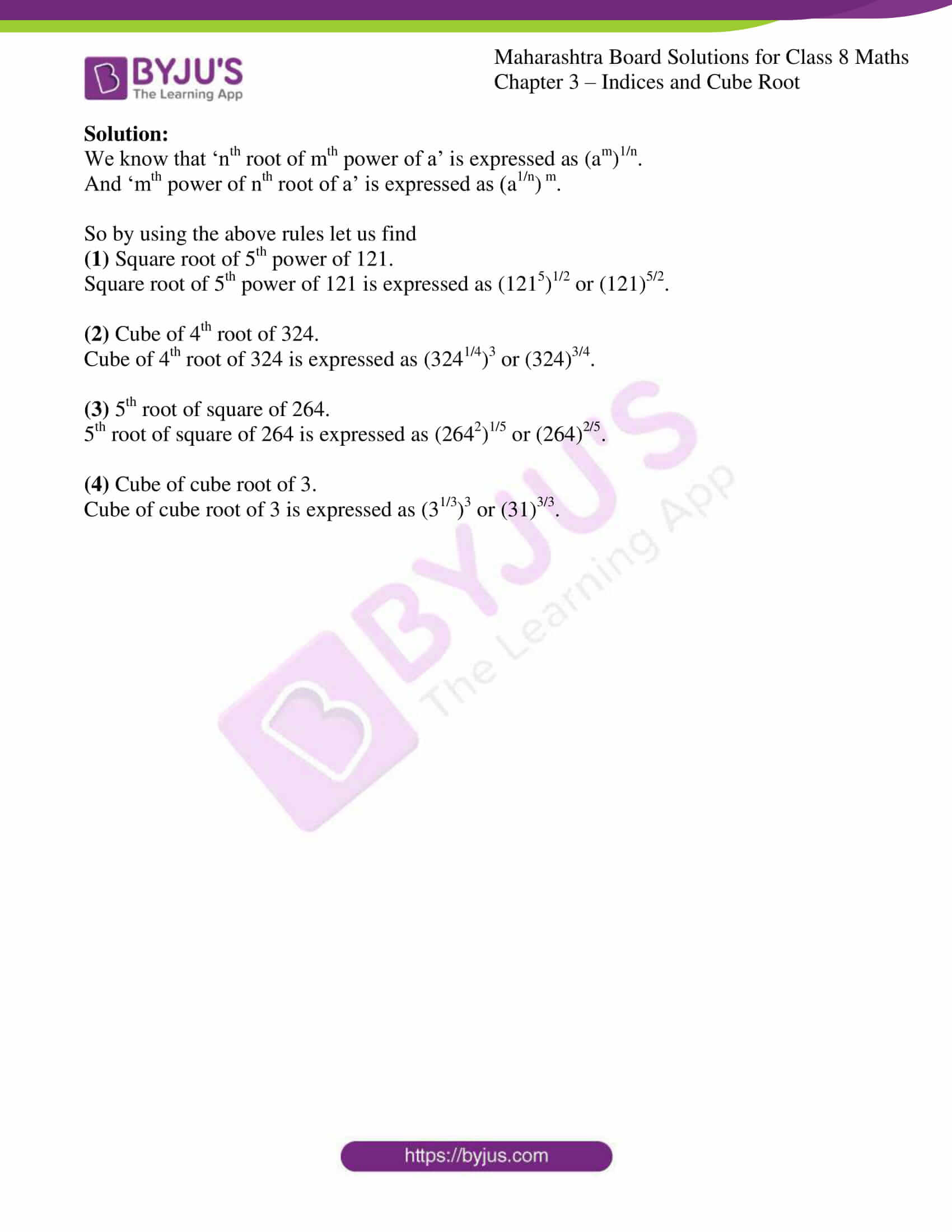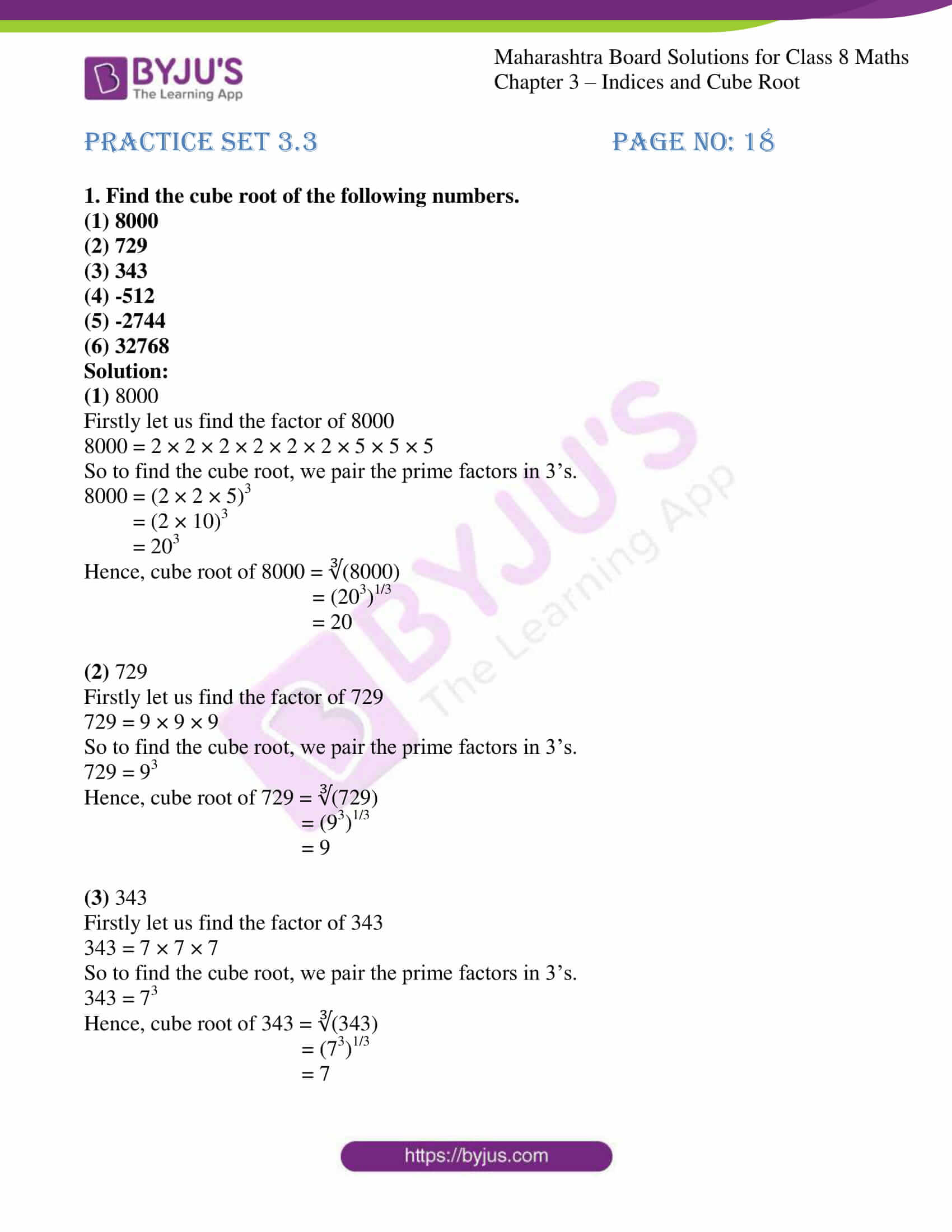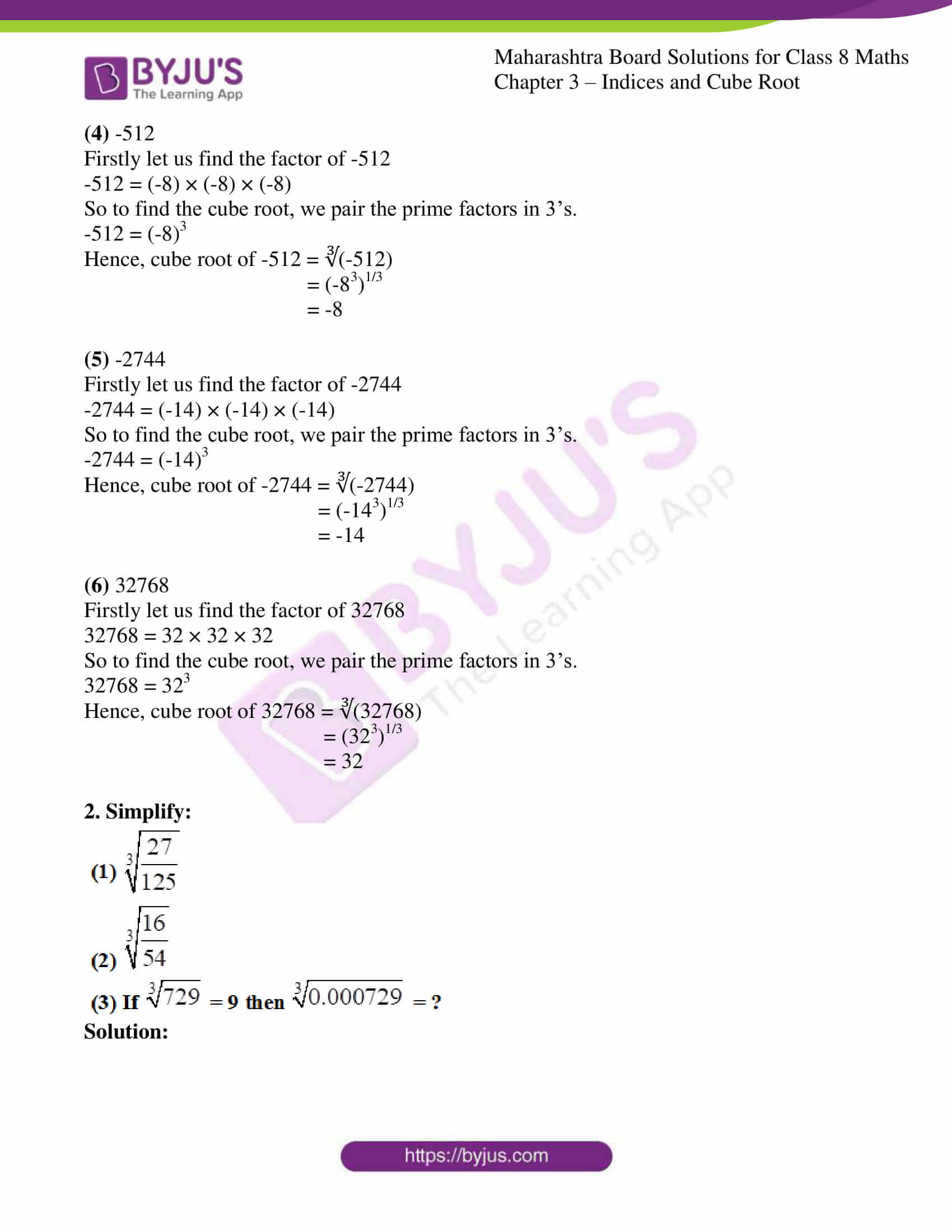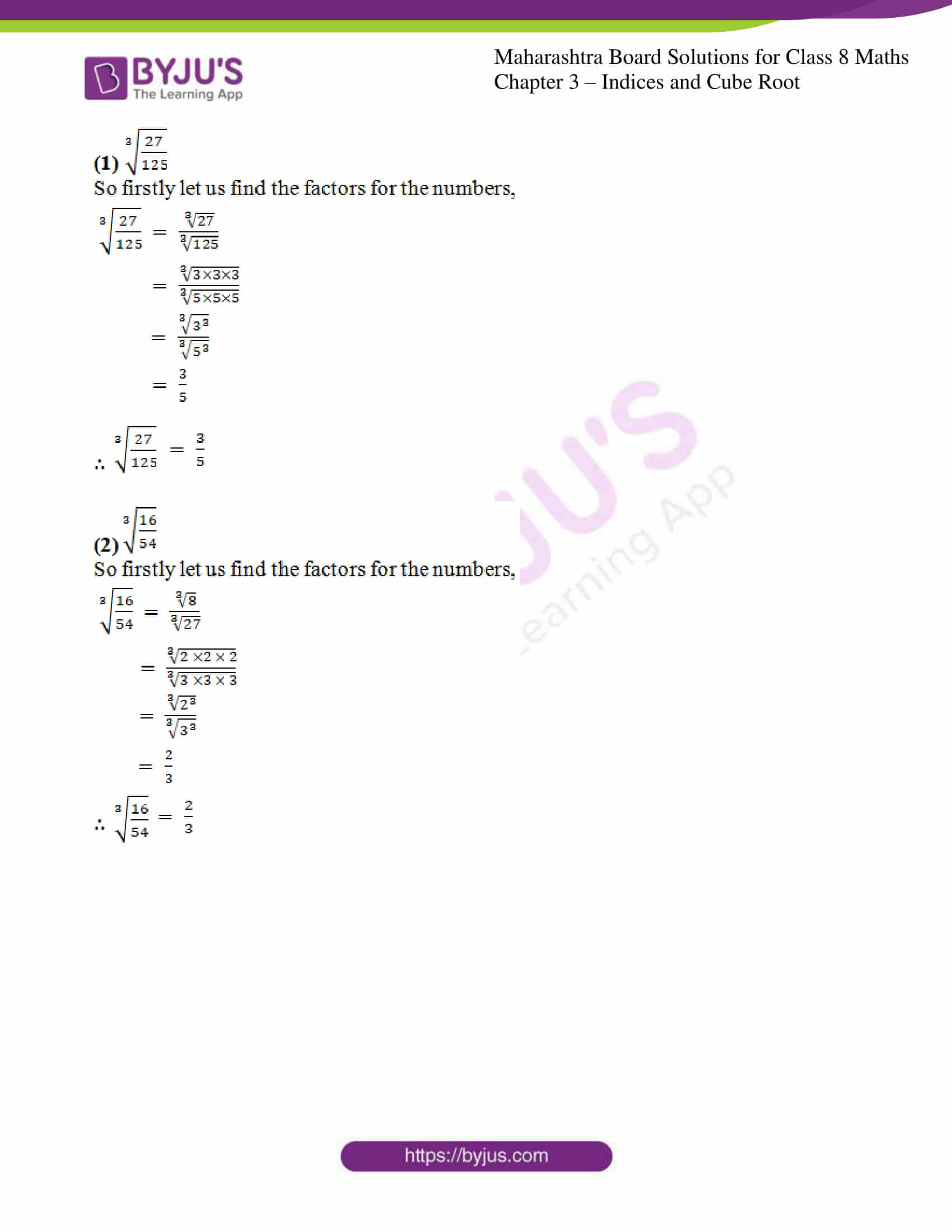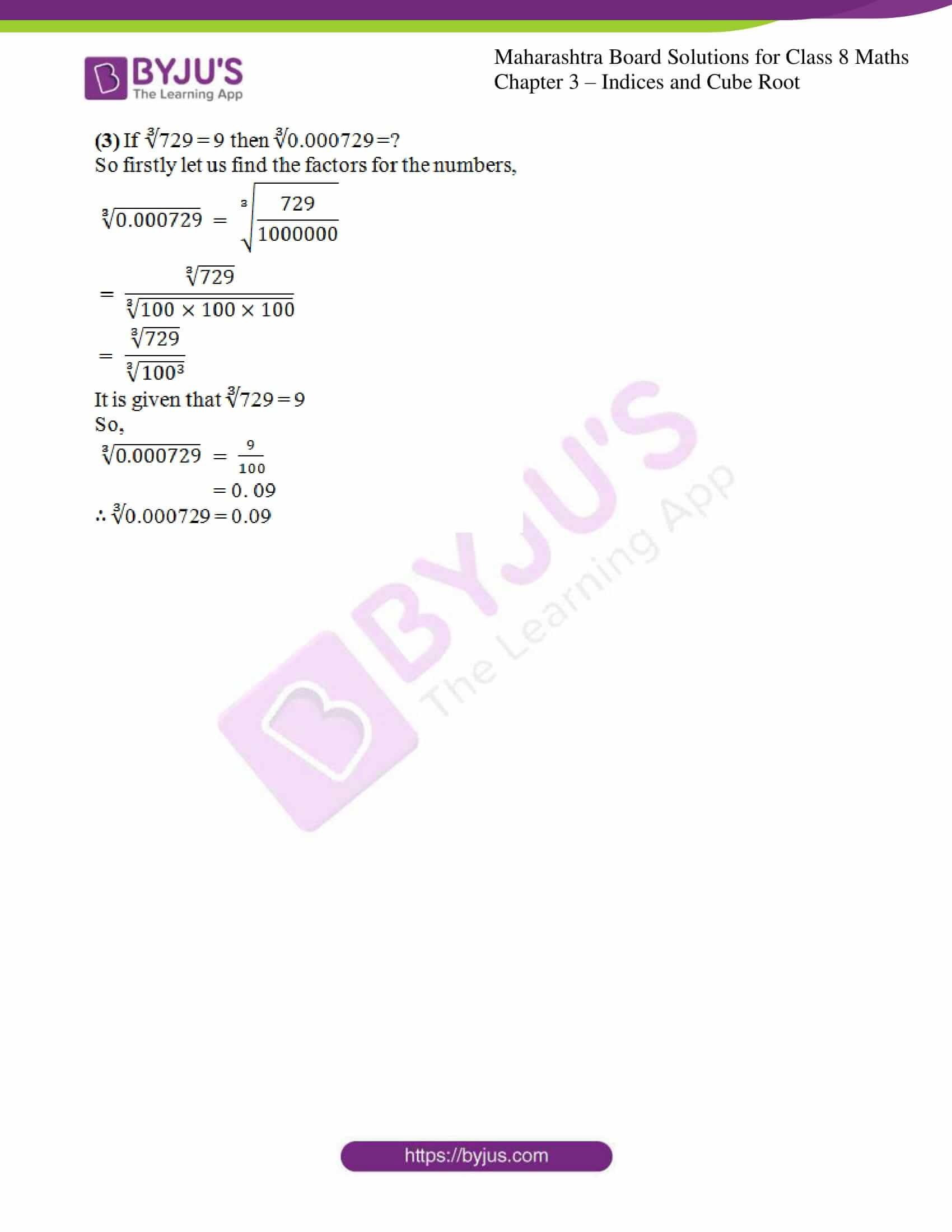### Access answers to Maharashtra Board Solutions For Class 8 Maths Part 1 Chapter 3- Indices and Cube Root.

Practice set 3.1 PAGE NO: 15

1. Express the following numbers in index form.
(1) Fifth root of 13
(2) Sixth root of 9
(3) Square root of 256
(4) Cube root of 17
(5) Eighth root of 100
(6) Seventh root of 30

Solution:

In general, nth root of ‘a’ is expressed as a1/n. where, a is the base and 1/5 is the index.

So now,

(1) Fifth root of 13

Index form of fifth root of 13is expressed as 131/5.

(2) Sixth root of 9

Index form of sixth root of 9 is expressed as 91/6.

(3) Square root of 256

Index form of square root of 256 is expressed as 2561/2.

(4) Cube root of 17

Index form of cube root of 17 is expressed as 171/3.

(5) Eighth root of 100

Index form of eighth root of 100 is expressed as 1001/8.

(6) Seventh root of 30

Index form of seventh root of 30 is expressed as 301/7.

2. Write in the form ‘nth root of a’ in each of the following numbers.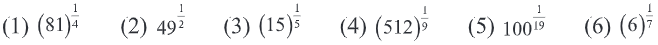Solution:

In general, a1/n is written as ‘nth root of a’.

So now,

(1) (81)1/4

(81)1/4 is written as ‘4th root of 81’.

(2) (49)1/2

(49)1/2 is written as ‘square root of 49’.

(3) (15)1/5

(15)1/5 is written as ‘5th root of 15’.

(4) (512)1/9

(512)1/9 is written as ‘9th root of 512’.

(5) (100)1/19

(100)1/19 is written as ‘19th root of 100’.

(6) (6)1/7

(6)1/7 is written as ‘7th root of 6’.

Practice set 3.2 PAGE NO: 16

1. Complete the following table.

 Sr. No. Number Power of the root Root of the power (1) (225)3/2 Cube of square root of 225 Square root of cube of 225 (2) (45)4/5 (3) (81)6/7 (4) (100)4/10 (5) (21)3/7

Solution:

Generally we can express the number am/n as

am/n = (am)1/n means ‘nth root of mth power of a’.

am/n = (a1/n)m means ‘mth power of nth root of a’.

So by using the above rules let us fill the table:

 Sr. No. Number Power of the root Root of the power (1) (225)3/2 Cube of square root of 225 Square root of cube of 225 (2) (45)4/5 Fourth power of fifth root of 45 Fifth root of fourth power of 45 (3) (81)6/7 Sixth power of seventh root of 81 Seventh root of sixth power of 81 (4) (100)4/10 Fourth power of tenth root of 100 Tenth root of fourth power of 100 (5) (21)3/7 Cube of seventh root of 21 Seventh root of cube of 21

2. Write the following number in the form of rational indices.
(1) Square root of 5th power of 121.
(2) Cube of 4th root of 324.
(3) 5th root of square of 264.
(4) Cube of cube root of 3.

Solution:

We know that ‘nth root of mth power of a’ is expressed as (am)1/n.

And ‘mth power of nth root of a’ is expressed as (a1/n) m.

So by using the above rules let us find

(1) Square root of 5th power of 121.

Square root of 5th power of 121 is expressed as (1215)1/2 or (121)5/2.

(2) Cube of 4th root of 324.

Cube of 4th root of 324 is expressed as (3241/4)3 or (324)3/4.

(3) 5th root of square of 264.

5th root of square of 264 is expressed as (2642)1/5 or (264)2/5.

(4) Cube of cube root of 3.

Cube of cube root of 3 is expressed as (31/3)3 or (31)3/3.

Practice set 3.3 PAGE NO: 18

1. Find the cube root of the following numbers.

(1) 8000

(2) 729

(3) 343

(4) -512

(5) -2744

(6) 32768

Solution:

(1) 8000

Firstly let us find the factor of 8000

8000 = 2 × 2 × 2 × 2 × 2 × 2 × 5 × 5 × 5

So to find the cube root, we pair the prime factors in 3’s.

8000 = (2 × 2 × 5)3

= (2 × 10)3

= 203

Hence, cube root of 8000 = ∛(8000)

= (203)1/3

= 20

(2) 729

Firstly let us find the factor of 729

729 = 9 × 9 × 9

So to find the cube root, we pair the prime factors in 3’s.

729 = 93

Hence, cube root of 729 = ∛(729)

= (93)1/3

= 9

(3) 343

Firstly let us find the factor of 343

343 = 7 × 7 × 7

So to find the cube root, we pair the prime factors in 3’s.

343 = 73

Hence, cube root of 343 = ∛(343)

= (73)1/3

= 7

(4) -512

Firstly let us find the factor of -512

-512 = (-8) × (-8) × (-8)

So to find the cube root, we pair the prime factors in 3’s.

-512 = (-8)3

Hence, cube root of -512 = ∛(-512)

= (-83)1/3

= -8

(5) -2744

Firstly let us find the factor of -2744

-2744 = (-14) × (-14) × (-14)

So to find the cube root, we pair the prime factors in 3’s.

-2744 = (-14)3

Hence, cube root of -2744 = ∛(-2744)

= (-143)1/3

= -14

(6) 32768

Firstly let us find the factor of 32768

32768 = 32 × 32 × 32

So to find the cube root, we pair the prime factors in 3’s.

32768 = 323

Hence, cube root of 32768 = ∛(32768)

= (323)1/3

= 32

2. Simplify: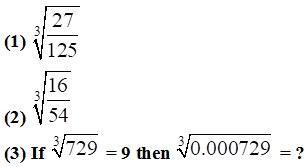Solution: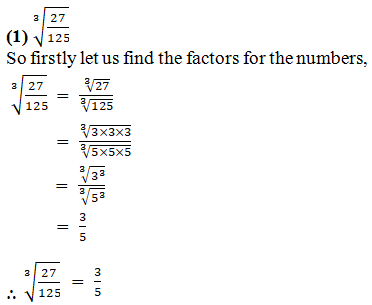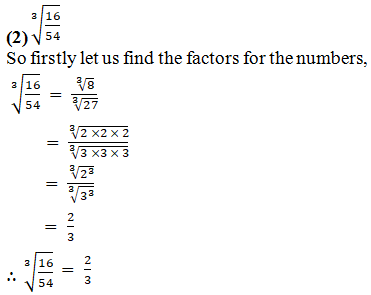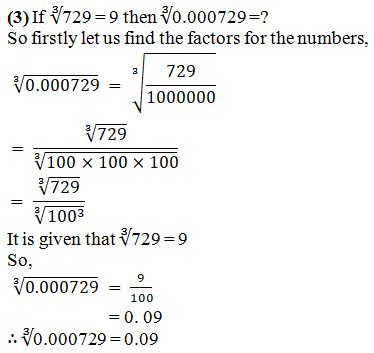Cube roots are used in day to day Mathematics, such as in powers and exponents or to find the side of a three-dimensional cube when its volume is given. Here, many such exercise problems are given, students can practice the solutions to secure good marks in the exams. Students can depend on these Solutions to understand all the topics under this chapter completely. Stay tuned to learn more about Indices and Cube Root, MSBSHSE Exam pattern and other information.

## Frequently Asked Questions on Maharashtra State Board Solutions for Class 8 Maths Chapter 3 Indices and Cube Root

### Where can I get Maharashtra State Board Class 8 Maths Solutions Chapter 3 Indices and Cube Root?

We have given the solutions as a scrollable PDF, and also mentioned a clickable link for the students to access the PDF. Meanwhile, the questions and the solutions  are also made available online on our webpage.

### Is Maharashtra State Board Class 8 Maths Solutions Chapter 3 Indices and Cube Root important?

Yes, these solutions are significant because they provide step-by-step solution or answer for the questions from the Chapter 3 of the Maharashtra state board Class 8 Maths. These solutions cover the topics that forms the basis for the questions that are likely to be asked in the board exams. Preparing with the help of these solutions can help students to score good marks in the exams.

### How do I study Maharashtra State Board Class 8 Maths Solutions Chapter 3?

Students are urged to first solve the questions and then to refer back to the solutions in order to identify the mistakes and solve them early on, so that it can be avoided for the exams. Timing the process helps to manage time better. This is also a good tool to analyse your exam preparations.